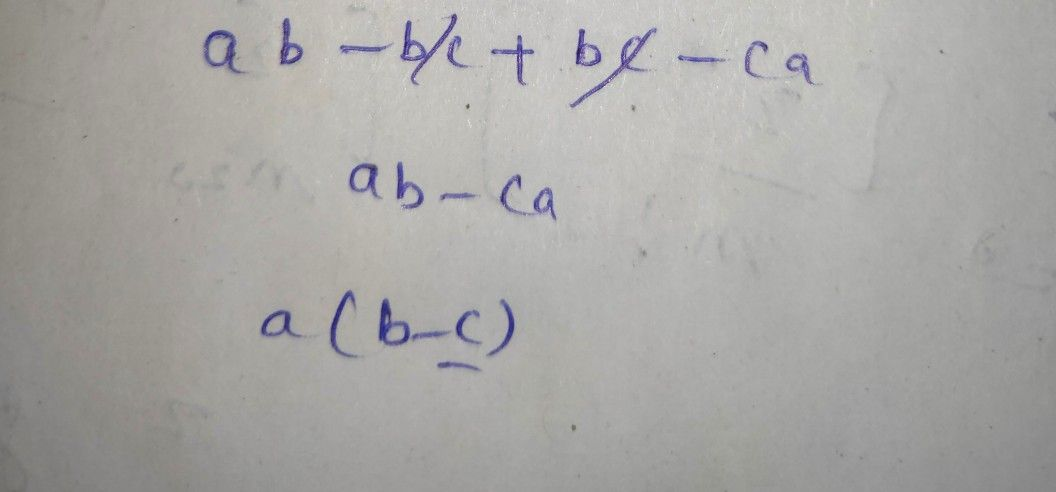Symbol
Problem$1$ $3-113$ $-1$ Learning Outcome) $=1$ $1,$ $ab-$ $bc$ Hi $bc-c0$ 0Ra $2$ $3x^{2}-2x+5xy-7$ uiel $8-9xy+3x^{2}-5x$ UE sl. $0$ $2$
Other
Question content